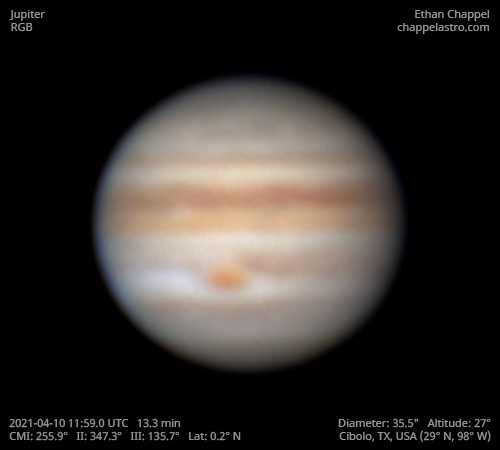# Jupiter 2021-04-10 11:59 UTC

CM1: 255.90°

CM2: 347.30°

CM3: 135.70°

CLat: +0.20°

Description

Although Jupiter has been visible in the morning sky for a while now, the wait for clear weather has finally ended. As the sky brightened, it was breezy and the air above was very turbulent. This was my first time having insulation wrapped around the OTA while imaging a planet. Despite having just bringing the scope out to a cooler environment, I saw no internal heat plume while collimating the secondary mirror.

This was also the first time I used my Chroma extended green filter on Jupiter. As expected, the new filter gave a brighter image compared to the standard green. The 11:59 image uses the standard green filter while the 12:00 image uses the extended green. I am hoping for better conditions soon so I can create a solid comparison between both filters.

The Equatorial Zone is still orange like it has been for the past 3 apparitions. The North Equatorial Belt is noticeably darker than the South Equatorial Belt.

Equipment

ZWO ASI290MM

Celestron EdgeHD 8

Logs
```FireCapture v2.7 03 BETA Settings
------------------------------------
Observer=Ethan Chappel
Location=Cibolo
Scope=Celestron C8 EdgeHD
Camera=ZWO ASI290MM
Filter=B
Profile=Jupiter
Diameter=35.43"
Magnitude=-2.11
CMI=259.1° CMII=350.4° CMIII=138.9°  (during mid of capture)
FocalLength=3250mm (F/16)
Resolution=0.19"
Filename=j2021-04-10_12-05-01_b_ec.ser
Date=2021_04_10
Start=12_03_46.703
Mid=12_05_01.706
End=12_06_16.709
Start(UT)=12_03_46.703
Mid(UT)=12_05_01.706
End(UT)=12_06_16.709
Duration=150.006s
Date_format=yyyy_MM_dd
Time_format=HH_mm_ss
LT=UT
Frames captured=11586
File type=SER
Binning=1x1
ROI=292x282
ROI(Offset)=1288x480
FPS (avg.)=77
Shutter=8.000ms
Gain=360 (60%)
AutoExposure=off
AutoGain=off
Brightness=1
HighSpeed=off
USBTraffic=50
Histogramm(min)=11
Histogramm(max)=184
Histogramm=72%
Noise(avg.deviation)=7.93
Limit=150 Seconds
Sensor temperature=78.8°F
FireCapture v2.7 03 BETA Settings
------------------------------------
Observer=Ethan Chappel
Location=Cibolo
Scope=Celestron C8 EdgeHD
Camera=ZWO ASI290MM
Filter=G
Profile=Jupiter
Diameter=35.42"
Magnitude=-2.11
CMI=254.7° CMII=346.1° CMIII=134.5°  (during mid of capture)
FocalLength=3350mm (F/16)
Resolution=0.18"
Filename=j2021-04-10_11-57-50_g_ec.ser
Date=2021_04_10
Start=11_56_35.393
Mid=11_57_50.396
End=11_59_05.400
Start(UT)=11_56_35.393
Mid(UT)=11_57_50.396
End(UT)=11_59_05.400
Duration=150.007s
Date_format=yyyy_MM_dd
Time_format=HH_mm_ss
LT=UT
Frames captured=11586
File type=SER
Binning=1x1
ROI=292x282
ROI(Offset)=1288x480
FPS (avg.)=77
Shutter=8.000ms
Gain=330 (55%)
AutoExposure=off
AutoGain=off
Brightness=1
HighSpeed=off
USBTraffic=50
Histogramm(min)=0
Histogramm(max)=150
Histogramm=58%
Noise(avg.deviation)=2.93
Limit=150 Seconds
Sensor temperature=78.8°F
FireCapture v2.7 03 BETA Settings
------------------------------------
Observer=Ethan Chappel
Location=Cibolo
Scope=Celestron C8 EdgeHD
Camera=ZWO ASI290MM
Filter=R
Profile=Jupiter
Diameter=35.42"
Magnitude=-2.11
CMI=252.5° CMII=343.9° CMIII=132.3°  (during mid of capture)
FocalLength=3350mm (F/16)
Resolution=0.18"
Filename=j2021-04-10_11-54-13_r_ec.ser
Date=2021_04_10
Start=11_52_58.526
Mid=11_54_13.523
End=11_55_28.521
Start(UT)=11_52_58.526
Mid(UT)=11_54_13.523
End(UT)=11_55_28.521
Duration=149.995s
Date_format=yyyy_MM_dd
Time_format=HH_mm_ss
LT=UT
Frames captured=11582
File type=SER
Binning=1x1
ROI=292x282
ROI(Offset)=1288x480
FPS (avg.)=77
Shutter=8.000ms
Gain=320 (53%)
AutoExposure=off
AutoGain=off
Brightness=1
HighSpeed=off
USBTraffic=50
Histogramm(min)=0
Histogramm(max)=146
Histogramm=57%
Noise(avg.deviation)=1.43
Limit=150 Seconds
Sensor temperature=79.0°F
```